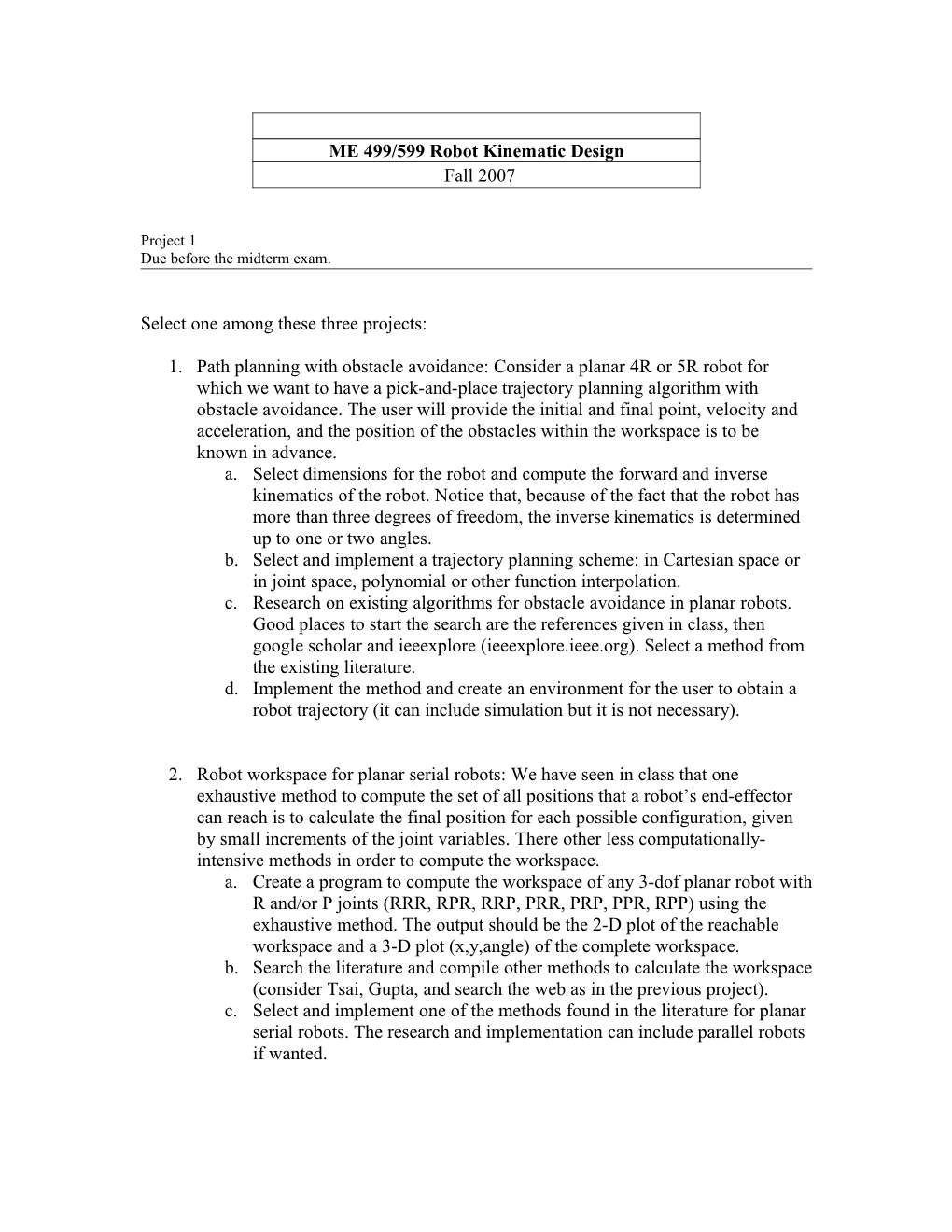# ME 499/599 Robot Kinematic Design## ME 499/599 Robot Kinematic Design

Fall 2007

Project 1

Due before the midterm exam.

Select one among these three projects:

1. Path planning with obstacle avoidance: Consider a planar 4R or 5R robot for which we want to have a pick-and-place trajectory planning algorithm with obstacle avoidance. The user will provide the initial and final point, velocity and acceleration, and the position of the obstacles within the workspace is to be known in advance.
2. Select dimensions for the robot and compute the forward and inverse kinematics of the robot. Notice that, because of the fact that the robot has more than three degrees of freedom, the inverse kinematics is determined up to one or two angles.
3. Select and implement a trajectory planning scheme: in Cartesian space or in joint space, polynomial or other function interpolation.
4. Research on existing algorithms for obstacle avoidance in planar robots. Good places to start the search are the references given in class, then google scholar and ieeexplore (ieeexplore.ieee.org). Select a method from the existing literature.
5. Implement the method and create an environment for the user to obtain a robot trajectory (it can include simulation but it is not necessary).
1. Robot workspace for planar serial robots: We have seen in class that one exhaustive method to compute the set of all positions that a robot’s end-effector can reach is to calculate the final position for each possible configuration, given by small increments of the joint variables. There other less computationally-intensive methods in order to compute the workspace.
2. Create a program to compute the workspace of any 3-dof planar robot with R and/or P joints (RRR, RPR, RRP, PRR, PRP, PPR, RPP) using the exhaustive method. The output should be the 2-D plot of the reachable workspace and a 3-D plot (x,y,angle) of the complete workspace.
3. Search the literature and compile other methods to calculate the workspace (consider Tsai, Gupta, and search the web as in the previous project).
4. Select and implement one of the methods found in the literature for planar serial robots. The research and implementation can include parallel robots if wanted.
1. Synthesis optimization for planar open chains with less than three degrees of freedom: Robots that have less degrees of freedom than the space they move on are called constrained robots. In the plane, there are just a few of these: R robot, RP, PR, and RR robots. The finite-position dimensional synthesis problem gives a finite number of solutions, as robots that pass exactly through the specified positions. The number of exact positions that can be defined depends on the topology of the robot and it is limited to a few (for instance, 5 positions for the RR robot or 4 positions for the RP robot). Sometimes we may want to have more control, and design a robot for a full trajectory of the end-effector.
2. State the kinematics equations in matrix form and in Clifford algebra form for the constrained planar serial robots.
3. Implement the solution for the finite-position synthesis of these robots (see Perez).
4. Research the literature for methods and algorithms to optimize the synthesis solution for a trajectory of the end-effector. See the reference books for traditional methods, Ge for the use of Clifford algebras, research the web for additional information.
5. Implement an optimization method for the given planar serial robots. The input for the system will be the trajectory of the end-effector and the desired type of robot, and the output will be the dimensions of the robot that most closely follows the trajectory. A simulation can be included.